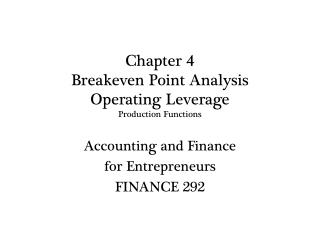DownloadDownload PresentationChapter 4 Breakeven Point Analysis Operating Leverage Production Functions

# Chapter 4 Breakeven Point Analysis Operating Leverage Production Functions

Télécharger la présentation## Chapter 4 Breakeven Point Analysis Operating Leverage Production Functions

- - - - - - - - - - - - - - - - - - - - - - - - - - - E N D - - - - - - - - - - - - - - - - - - - - - - - - - - -

1. ### Chapter 4Breakeven Point AnalysisOperating Leverage Production Functions

Accounting and Finance for Entrepreneurs FINANCE 292
2. Breakeven Point Analysis Job #1 in Managing Profitability When will the business breakeven? The minimum level of sales to cover your operating expenses Labor Materials Overhead Sales minus Operating Expenses = operating income Operating Income = zero at the BEP
3. Breakeven Point Analysis TR= Total Revenues TC = Total Costs = Variable + Fixed BEP where TR = TC When TR = TC, Operating Income = 0 Assumptions: When sales are 0, no VC are incurred As output increases from zero, VC accrue. Profits = TR> TC Losses = TR < TC
4. Breakeven Point Analysis Estimating the BEP When unit costs and Fixed Costs are known BEP = FC / (Price – Variable Cost) Example from Text: making Pizzas Suppose it costs \$2.60 to make, bake, slice, and hand to server each pizza (unit variable costs) Selling price = \$7.00 Fixed Overhead = \$7,500 per month.
5. Breakeven Point Analysis Making Pizzas (con’t) BEPunits = FC / (P – VC) BEPunits= 7,500 / (7 – 2.60) = 1705 pizzas BEP\$ = 1705 * 7 = \$11,935 in sales Joe’s Pizzeria need to make an average of 57 pizzas per day to cover his fixed overhead. If Joe can make 100 pizzas per day, the operating profit = \$5,700 per month or \$68,400 per year.
6. Breakeven Point Analysis How can you compute the BEP if you have many different products? BEP(\$) = FC / CM CM = Contribution Margin (the amount of each sales dollar left after paying variable costs to cover our fixed costs (FC) Coastal Marine Services (See Table 4.1) CMS generated \$115,000 in gross profit on sales of \$224,000. The CM = 115,000/224,000 = .5134
7. Table 4.1
8. Breakeven Point Analysis CMS Example (con’t) CM = .5134 51.34 cents of each dollar of sales is left after we cover the variable costs (= cost of goods sold) to “contribute” (CM) to covering our fixed costs (FC). BEP(\$) = FC / CM = \$46,000 / .5134 = \$90,436 CMS must generate \$90,436 in sales in order to breakeven. Once it passes that point, CMS begins top earn operating income.
9. Breakeven Point Analysis Contribution Margin, Contribution Margin Ratio, and Gross Profit Margin CMR = GPM CM is a dollar value CMR and GPM are percentages Accountants speak of contribution margin in much the same way that financial analysts takes about gross profit margin.
10. How Operating Leverage Impacts the BEP BEP and Operating Leverage Laying quietly in the background of BEP analysis is the business’s production function The mix of labor and capital used to produce the goods or services sold to its customers. How does the PF impact operating leverage? It relates variable and fixed costs to profitability as sales grow. How does O.L influence the BEP? The greater the O.L. the greater the impact on operating profitability.
11. OPERATING LEVERAGE(Operating Risk) A. Degree of Operating Leverage 1. Extent to which fixed costs are utilized in production 2. Businesses tend to increase their DOL over time. a. Streamlining production due to competition. b. Increased use of automation is the main strategy. c. Increased DOL means increased capital intensities (fixed costs). 3. Labor versus capital intensity; a. Labor intensive is usually associated with low DOL. b. Capital intensive with high DOL.
12. Degree of Operating Leverage The production of goods and services can be accomplished by labor intensive processes or by capital intensive processes. Labor Intensive: most of the work is done by hand Think building a house Capital Intensive: much of the work is done using machines Think steel or automobiles The graphic representation is seen below: Capital Intensive Labor Intensive
13. Degree of Operating Leverage B. High DOL associated with high break-even points. 1. Variable costs; high costs lead to low gross profits. Fixed costs; high costs lead to low operating income. Important note: We do not include depreciation expense in the fixed expense totals when computing cash flow break-even point. The reason should be obvious; depreciation is a non-cash expense. BEP analysis is a cash flow concept.
14. Chapter Break
15. Estimating the Degree of Operating Leverage Using Income Statement data DOL = Gross Profit / Operating Income DOL = 2.00 means for each \$2.00 in GP, we generate \$1 in O.I.
16. Changes in DOL Unrelated to Production Function Changes DOL is affected by changes in; the production function Changes in the level of sales Changes in overhead expenses unrelated to the production function. What happens if sales increase? DOL of 1.67 implies that it takes \$1.67 of gross profits to produce \$1.00 of operating income
17. Impact of an Increase in Overheadon the DOL The DOL is also sensitive to autonomous changes in overhead expenses. The increase in overhead could be mistaken for a change in the operating leverage of the business.
18. Impact of a Change in the Production Function What happens when businesses increase their capital intensity? ~ A Change in the Production Function (DPF)